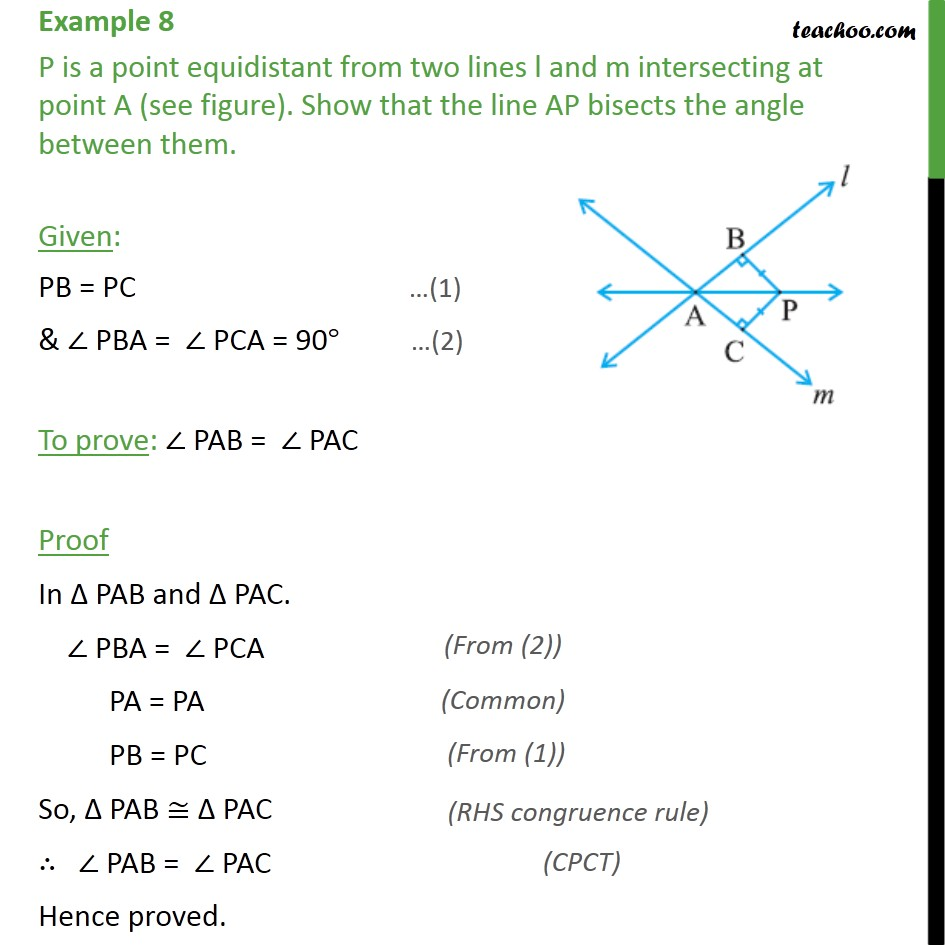1. Chapter 7 Class 9 Triangles (Term 1)
2. Serial order wise
3. Examples

Transcript

Example 8 P is a point equidistant from two lines l and m intersecting at point A (see figure). Show that the line AP bisects the angle between them. Given: PB = PC & PBA = PCA = 90 To prove: PAB = PAC Proof In PAB and PAC. PBA = PCA PA = PA PB = PC So, PAB PAC PAB = PAC Hence proved.

Examples